# 目标检测和感受野的总结和想法¶

### 1. 概念¶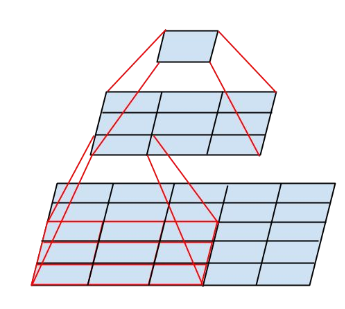1. 并不是感受野内所有像素对输出向量的贡献相同，实际感受野是一个高斯分布，有效感受野仅占理论感受野的一部分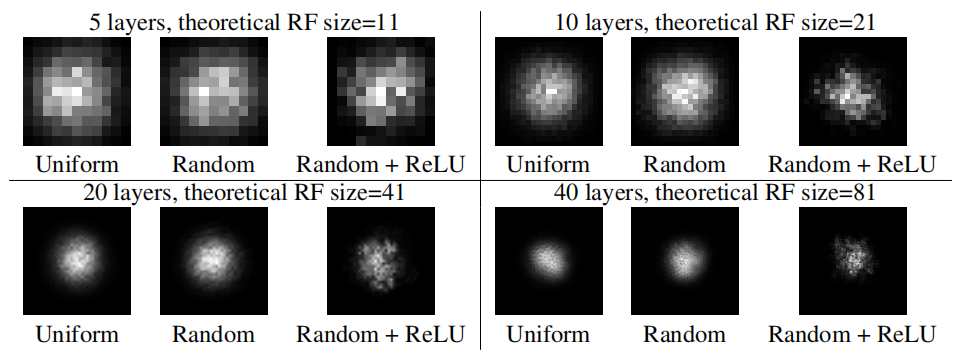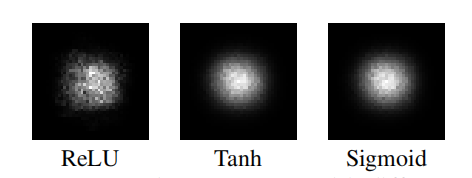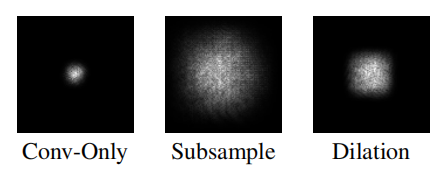2. 另外就是非常关心的问题，实际感受野是如何变化的？实际感受野和理论感受野的关系是什么样的？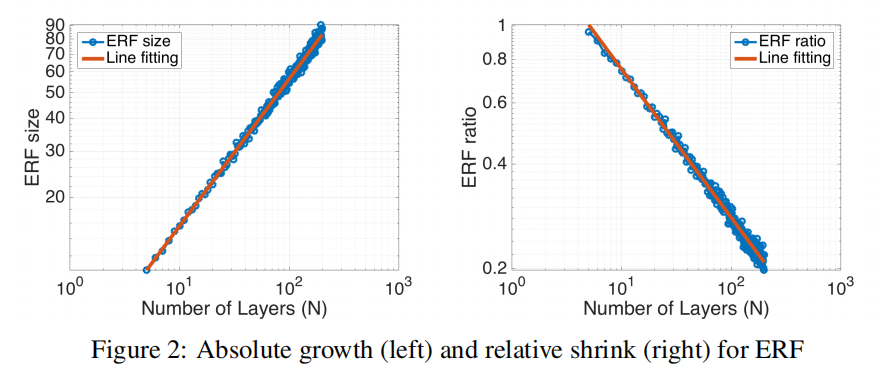3. 训练的过程中，感受野也会发生变化。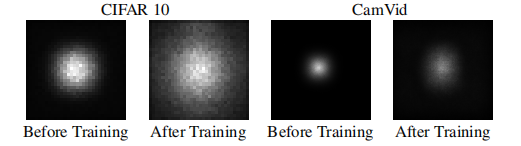### 2. 计算¶

#### 2.1 一种简单的感受野计算方法¶

• 第一个feature map(也就是原始图片)的感受野默认为1

• 经过kernelSize=k, s=1 的卷积层, 感受野计算方法如下:

r = r +(k-1)
• 经过kernelSize=k, s=2 的卷积层, 感受野计算方法如下：
r = (r\times2)+(k-2)
• 经过s=2的maxpool或avgpool层,感受野计算方法如下：
r = r \times 2
• 经过kernelSize=1的卷积层不改变感受野
• 经过全连接层和Global Average Pooling层的感受野就是整个输入图像
• 经过多路分支的网络，按照感受野最大支路计算
• shortcut层不改变感受野
• ReLU、BN、Dropout不改变感受野
• 全局Stride等于所有层Stride的累乘
P=\frac{(f_{out}-1)\times stride -f_{in}+ kernelSize}{2}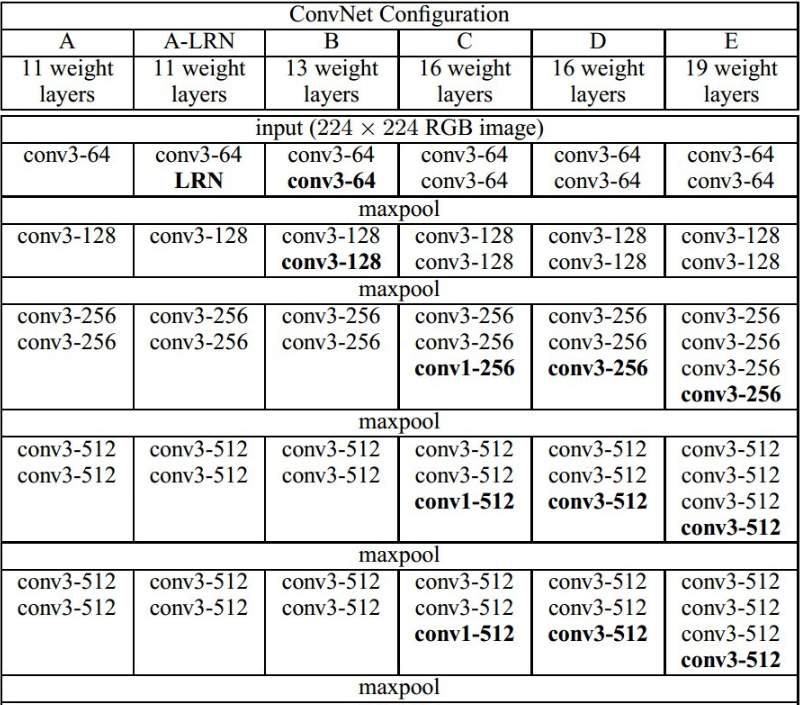VGG16对应D系列，来计算这一列的感受野（从上往下）：

R=(((((1+2+2)x2+2+2)x2+2+2+2)x2+2+2+2)x2+2+2+2)x2=308
S=2x2x2x2x2=32
P=138


PS:提供一下pytorch和tensorflow计算感受野的库：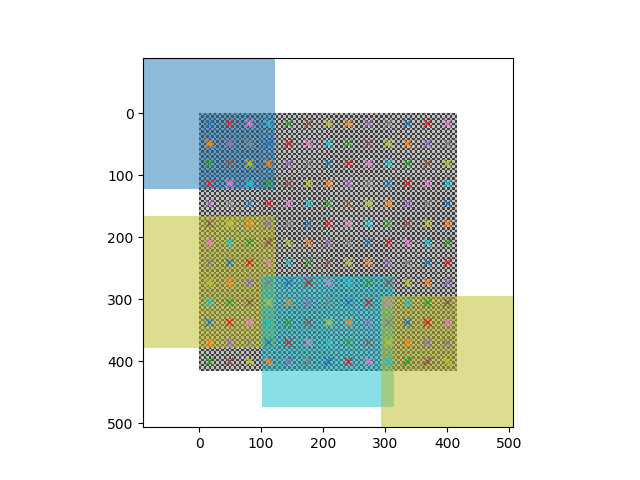#### 2.2 自下而上的计算方法¶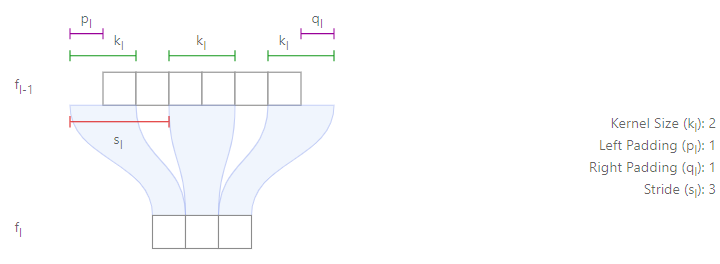$l$代表第几层；$f$代表feature map；$p$代表left padding；

$q$代表right padding；$s$代表stride，$k$代表kernel size。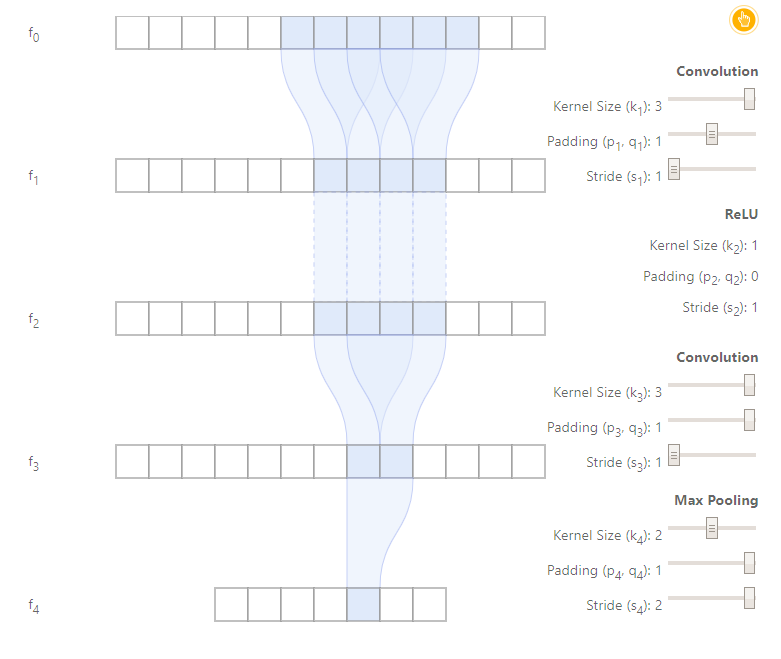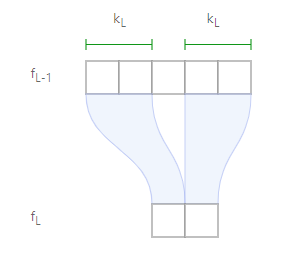r_{l-1}=s_l\times r_l+(k_l-s_l)

r_0=\sum_{l=1}^{L}((k_l-1)\prod_{i=1}^{l-1}s_i)+1

Effective Stride:

S_{l-1}=s_l\times S_l

S_l=\prod_{i=l+1}^{L}s_i

P_{l-1}=s_l\times P_l+p_l

P_l=\sum_{m=l+1}^{L}p_m\prod^{m-1}_{i=l+1}s_i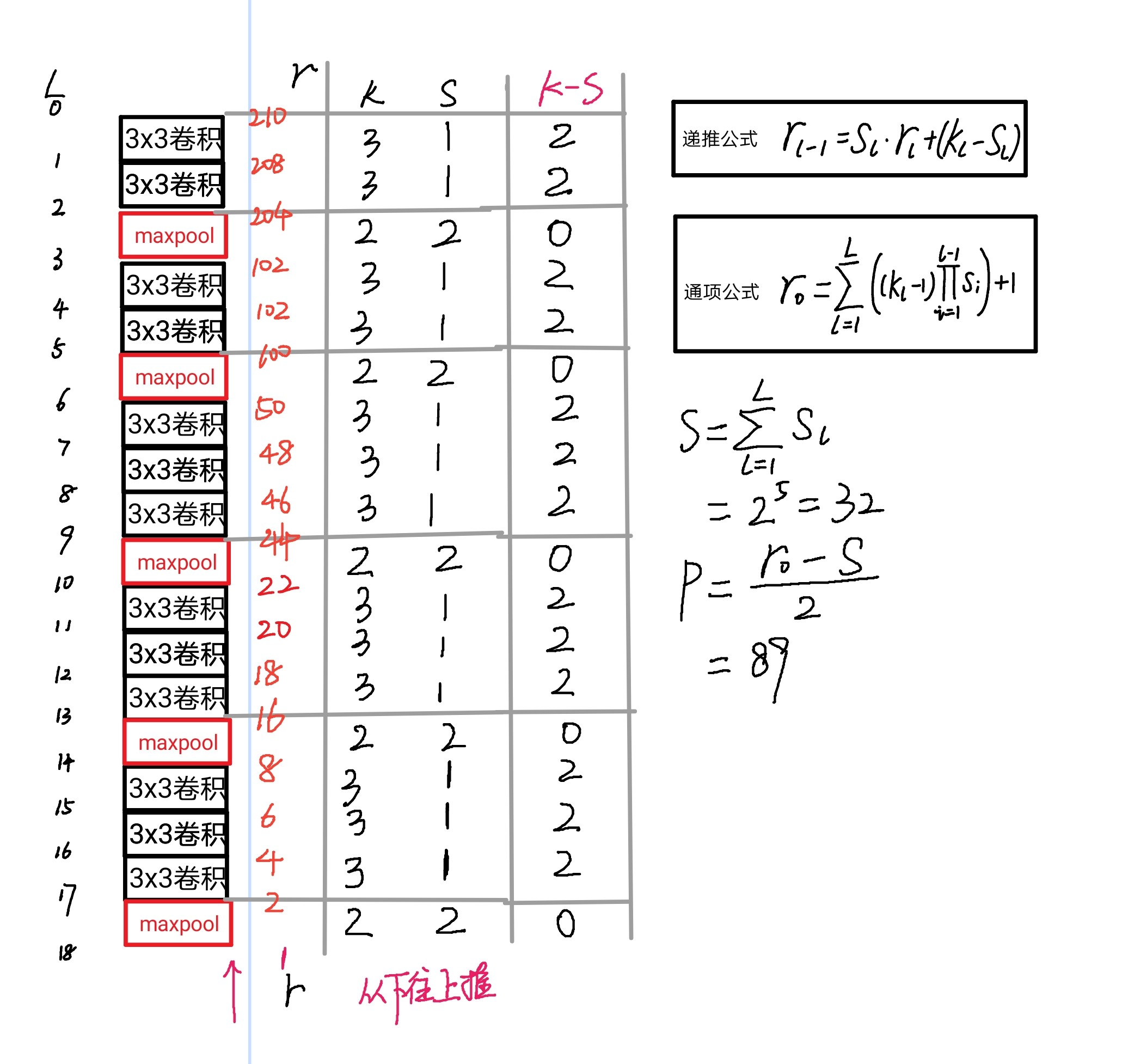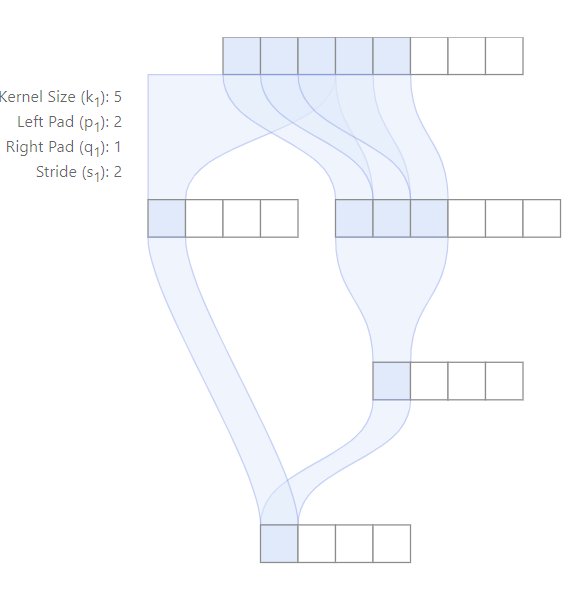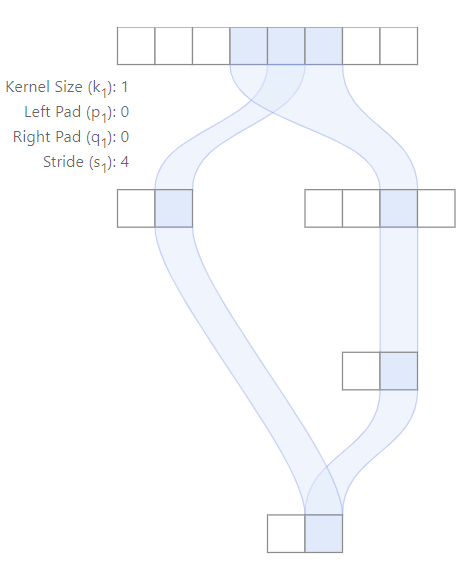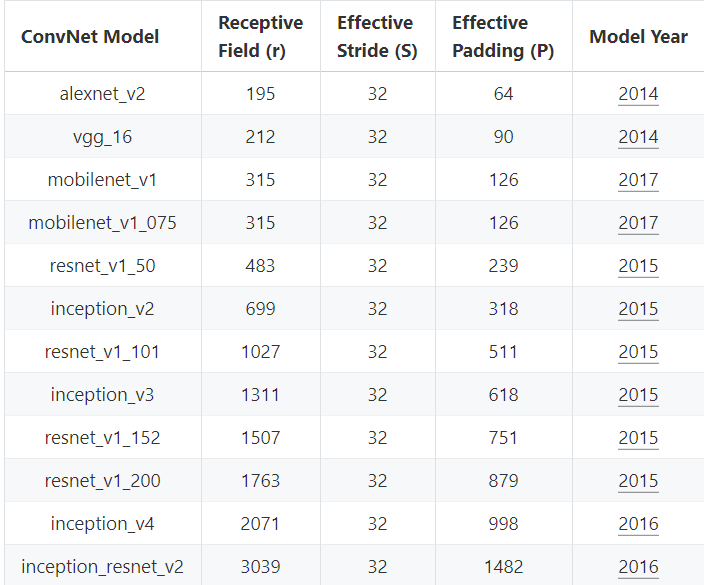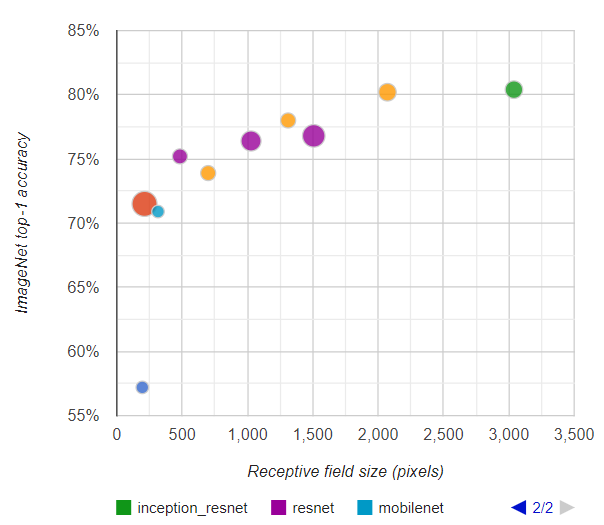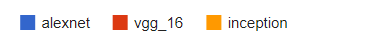• 空洞卷积：引入空洞率$\alpha$, 经过空洞卷积，kernel size有原来的$k$变为$\alpha \times (k-1)+1$,所以只要替换原来的公式中的k即可。
• 上采样：一般通过插值来实现，假如$\alpha$通过2倍上采样得到$\beta$，那么认为这个层感受野计算等价于与$\alpha$的kernel size相等的卷积。(这个地方不是特别理解，按照自己想的，一般upsample不会改变感受野，下边是谷歌论文中关于这部分的讲解，希望能读懂的大佬能联系一下我，我的联系方式在文末)

Upsampling. Upsampling is frequently done using interpolation (e.g., bilinear, bicubic or nearest-neighbor methods), resulting in an equal or larger receptive field — since it relies on one or multiple features from the input. Upsampling layers generally produce output features which depend locally on input features, and for receptive field computation purposes can be considered to have a kernel size equal to the number of input features involved in the computation of an output feature.

• 可分离卷积: 等价于普通卷积，并没有变化。
• BatchNorm:推理过程中BN不改变感受野，而训练过程中，BN的感受野是整个输入图像。
• 转置卷积: 会增加感受野，和普通计算方法类似

### 3. 作用¶

1.目标检测：像SSD、RPN、YOLOv3等都使用了anchor，而anchor的设计正是依据感受野，如果感受野太小，只能观察到局部的特征，不足以得到整个目标的信息。如果感受野过大，则会引入过多噪声和无效信息。Anchor太大或太小均会影响性能。

2.语义分割：最终预测的像素的感受野越大越好，涉及网络一般也是越深越好，这样才能捕获更多的上下文信息，预测才会更准。

3.分类任务：图像分类中最后卷积层的感受野要大于输入图像，网络深度越深，感受野越大，性能越好。

### 4. 关系¶

Anchor一般是通过先验进行指定的，Anchor与目标的大小和位置越匹配(IOU>0.5or0.7)，回归效果就会越好。如果小人脸和anchor的IOU过小，就会造成anchor很难回归到GT上，从而导致Recall过低。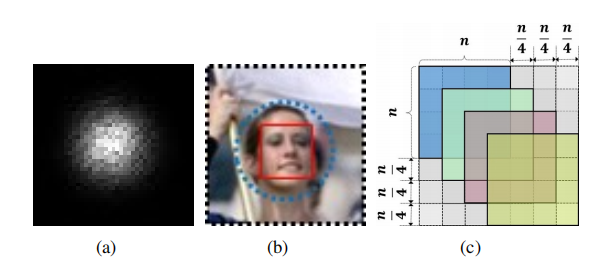Anchor大小<实际感受野<理论感受野

SFD是基于SSD进行改进的，SSD中使用了6个层来进行检测，以下是文章中设置内容: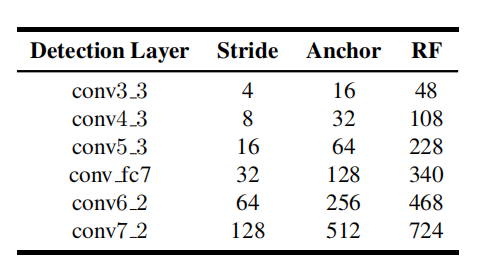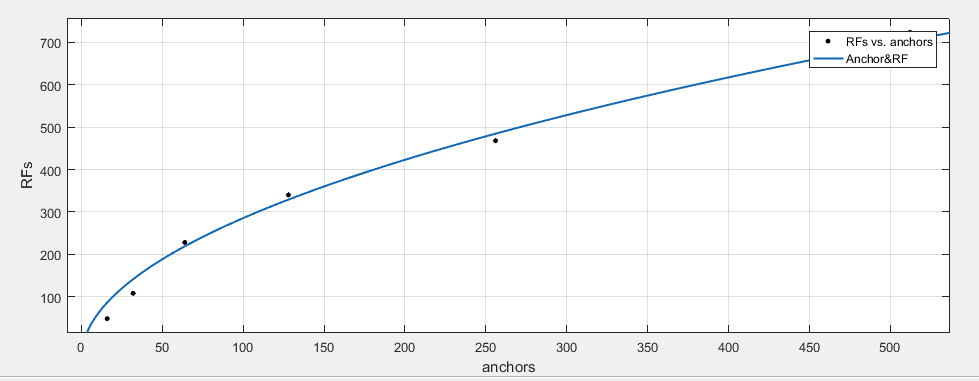y=9.421\times \sqrt{12.44\times x}-46.92 \\ R^2=0.989

SFD效果可以说是非常好了，以下是mAP对比图：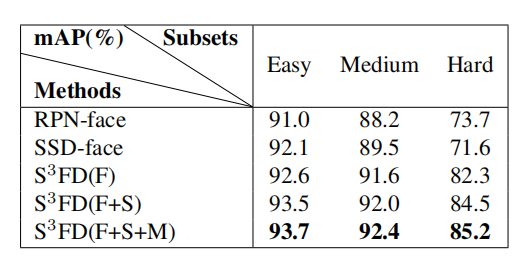### 5. 总结¶

1. 首先，许多经典的网络结构中的这些卷积不是随便决定的，在修改网络的时候不能随便删除。比如在yolov3中，backbone后边的几个卷积再接yolo层这个当时就觉得很随意，但是他是有一定道理的。

2. 假如出于对小目标的考虑，想要降低下采样率，那么直接删掉一个maxpool层，或者删掉stride=2的卷积层都是不可取的。通过公式我们知道，stride对一个网络的感受野影响非常大，而且会对后边的网络层的感受野都进行影响，所以要通过使用stride=1的maxpool或者空洞卷积来弥补对感受野带来的损失。

3. 实际感受野依然是一个超参数，他是会随着训练的过程发生变化，我们无法准确计算出来实际感受野，但是通过分析anchor，实际感受野和理论感受野，我们知道了anchor<实际感受野<理论感受野，所以anchor还是会被理论感受野的大小所限制。

4. 自己尝试过使用kmeans算法聚类自己数据集的Anchor，之前两个数据，两次聚类，都出现了自己聚类得到的Anchor不如默认的Anchor得到的结果。之前一直不知道原因在哪里。在总结了感受野以后，我觉得可以合理推测，Anchor应该和实际感受野尽量匹配，但是实际感受野实际上是一个超参数，通过聚类得到的anchor有一定代表性，但是效果反而不如默认的anchor，这是因为我们自己聚类得到的Anchor的范围实际上没有默认的广。

比如yolov3-tiny里边的anchor最小是【10，14】最大是【344，319】,经过计算理论感受野是382,按照SFD里边推导得到的公式计算，对应的anchor大小应该为159，如果按照理论感受野的三分之一来计算，大概是127.3。大概在这个范围，自己聚类得到的最大anchor也就是20左右，所以网络如果想要适应（回归）20左右的anchor需要网络更长时间的训练。

（1）S3FD

（2）Computing Receptive Fields of Convolutional Neural Networks

（3）Understanding the Effective Receptive Field in Deep Convolutional Neural Networks